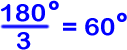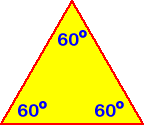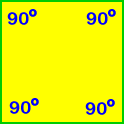The sum of the measures of the interior angles of a triangle is 180 degrees.

Since all the sides of equilateral triangles are the same length, all the angles are the same...The interior angles of an equilateral triangle are all 60 degrees.

That's easy! By definition, all the interior angles of a square are right angles -- That means that they are all 90 degrees.